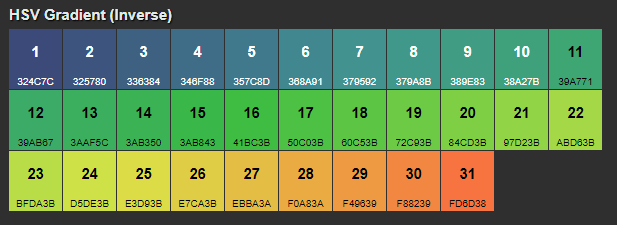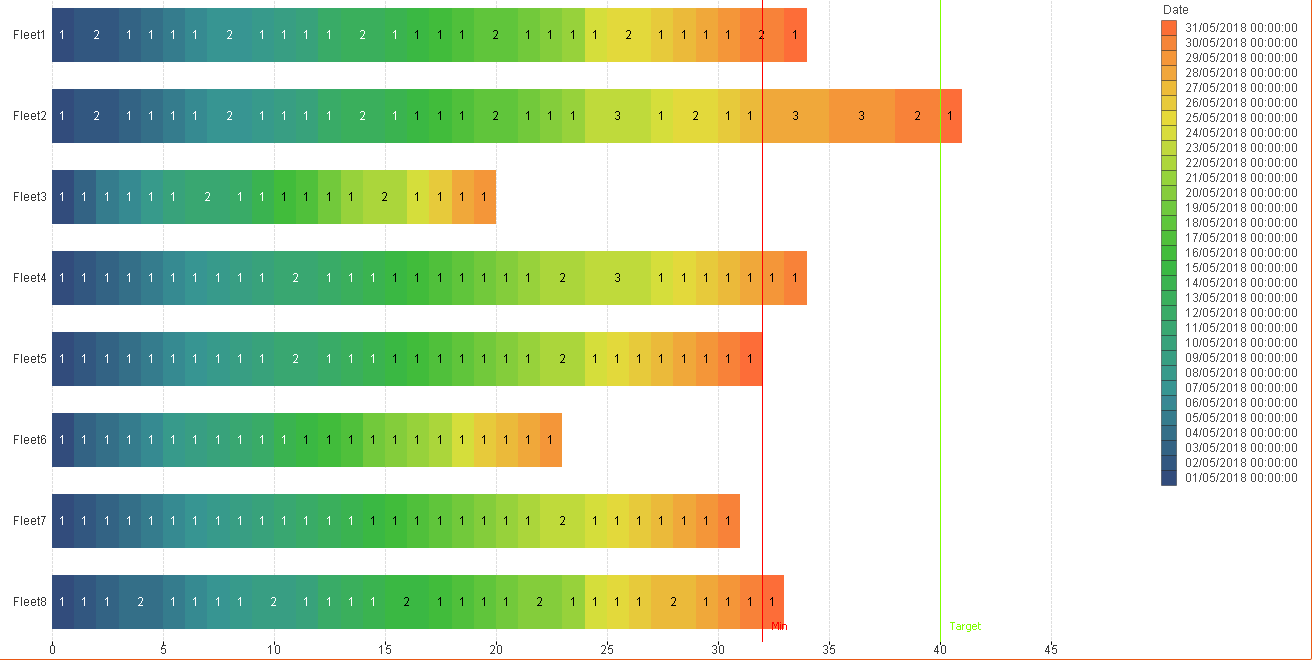# QlikView App Dev

Discussion Board for collaboration related to QlikView App Development.

Announcements
cancel
Showing results for
Did you mean:Contributor II

## Gradient fill stacked bar chart where Dimension is Day of month

Hello.

I have been asked to mimic and existing report for a new customer.

I'm documenting the solution I used here but i'm interested in Qlik experts critique and also enhancements.

I'm working from test data at the moment.

The issue is that they have a series of services (each bar) and they want to see how much each service is being used per day in a given month.

The bar chart is fairly simple, the dimensions are

A) the service (fleet 1..n)

B) the date field from the calendar

the expression (%JOIN_CALENDAR)

c) Count (Distinct workitems)

The issue is that the default colours loop after 15.

The clients corporate colours re white, blue and orange, so i used colour picker to get the # hex for their blue and orange and put it into a colour gradient generator to make a nice colour gradient with 31 steps.I copied the data across into excel and used the formula =HEX2DEC(MID(C1,1,2)) to split it, and then used

="SET v_Colour_"&A1&" = 'RGB(" & E1& ", " & F1&", "&G1&")';" to automate the variables.

e.g.

SET v_Colour_1 = 'RGB(50, 76, 124)';

I placed the variables in the load script, looking at it now i should have been let not set?

in the expression i set the background colour as follows,

=if(Day(%JOIN_CALENDAR)='1',\$(v_Colour_1), if(Day(%JOIN_CALENDAR)='2',\$(v_Colour_2),

if(Day(%JOIN_CALENDAR)='3',\$(v_Colour_3), if(Day(%JOIN_CALENDAR)='4',\$(v_Colour_4),

if(Day(%JOIN_CALENDAR)='5',\$(v_Colour_5), if(Day(%JOIN_CALENDAR)='6',\$(v_Colour_6),

if(Day(%JOIN_CALENDAR)='7',\$(v_Colour_7), if(Day(%JOIN_CALENDAR)='8',\$(v_Colour_8),

if(Day(%JOIN_CALENDAR)='9',\$(v_Colour_9), if(Day(%JOIN_CALENDAR)='10',\$(v_Colour_10),

if(Day(%JOIN_CALENDAR)='11',\$(v_Colour_11), if(Day(%JOIN_CALENDAR)='12',\$(v_Colour_12),

if(Day(%JOIN_CALENDAR)='13',\$(v_Colour_13), if(Day(%JOIN_CALENDAR)='14',\$(v_Colour_14),

if(Day(%JOIN_CALENDAR)='15',\$(v_Colour_15), if(Day(%JOIN_CALENDAR)='16',\$(v_Colour_16),

if(Day(%JOIN_CALENDAR)='17',\$(v_Colour_17), if(Day(%JOIN_CALENDAR)='18',\$(v_Colour_18),

if(Day(%JOIN_CALENDAR)='19',\$(v_Colour_19), if(Day(%JOIN_CALENDAR)='20',\$(v_Colour_20),

if(Day(%JOIN_CALENDAR)='21',\$(v_Colour_21), if(Day(%JOIN_CALENDAR)='22',\$(v_Colour_22),

if(Day(%JOIN_CALENDAR)='23',\$(v_Colour_23), if(Day(%JOIN_CALENDAR)='24',\$(v_Colour_24),

if(Day(%JOIN_CALENDAR)='25',\$(v_Colour_25), if(Day(%JOIN_CALENDAR)='26',\$(v_Colour_26),

if(Day(%JOIN_CALENDAR)='27',\$(v_Colour_27), if(Day(%JOIN_CALENDAR)='28',\$(v_Colour_28),

if(Day(%JOIN_CALENDAR)='29',\$(v_Colour_29), if(Day(%JOIN_CALENDAR)='30',\$(v_Colour_30),

if(Day(%JOIN_CALENDAR)='31',\$(v_Colour_31), RGB(0,0,0)) ) ) ) ) ) ) ) ) ) ) ) ) ) ) ) ) ) ) ) ) ) ) ) ) ) ) ) ) ))

This is the chart:So, what can I do to optimise the 31 stage Nested If in the expression?

Can I use a function to accept a day number and return a variable name or RGB() string?

If so, How?

3 RepliesSpecialist II

Hi,

you could put it in your master calendar during the load ?

regardsContributor II
Author

how so?

set up a day number inline table mapping load and use an applymap to link month day to the RGB code as DayColour... then just use the DayColour field direct in the backgroundcolour?Specialist II

yes I think so

or just a new 31 rows table with daynumber, rgb color

add daynumber in calendar if not present